# Equation of infinite order

(diff) ← Older revision | Latest revision (diff) | Newer revision → (diff)

in the complex domain

A differential equation of the formwhereis the unknown function of the complex variableand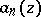,are given functions. Equations of infinite order which have been most thoroughly studied are those with constant coefficients: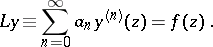If the characteristic function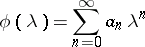is an entire function of exponential type, the left-hand side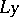makes sense forif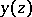is an analytic function in the disc,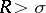. If, one must assume thatis an entire function. A difference from an equation of finite order consists already in the fact that the solutionmay have singular points even ifis an entire function. Ifandis an entire function, the domain of existence of a solution is convex . The general solution consists of a particular solution and the general solution of the corresponding homogeneous equation. Let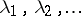be the roots of the characteristic equationand let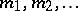be their respective multiplicities. The homogeneous equation has elementary particular solutions(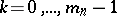;). The solution of the homogeneous equation can be written as a series of elementary particular solutions, formed according to a definite rule. If the characteristic functionhas regular growth (in a certain sense), it is possible to find a subsequence of the partial sums of this series converging to. In the general case the functionmay be approximated, as accurately as one pleases, by finite linear combinations of elementary solutions . If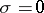, an equation of infinite order may have non-analytic solutions . Under certain conditions these solutions form a quasi-analytic class with weaker bounds on the growth of the derivatives than in the classical Denjoy–Carleman theorem (cf. Quasi-analytic class).

Equations of infinite order have various applications. They are used in the study of sequences of Dirichlet polynomials, completeness of systems of analytic functions, uniqueness of analytic and harmonic functions, and in solvability questions of analytic problems such as the generalized quasi-analyticity problem, the generalized uniqueness problem of moments, etc.

How to Cite This Entry:
Equation of infinite order. Encyclopedia of Mathematics. URL: http://encyclopediaofmath.org/index.php?title=Equation_of_infinite_order&oldid=16246
This article was adapted from an original article by A.F. Leont'ev (originator), which appeared in Encyclopedia of Mathematics - ISBN 1402006098. See original article